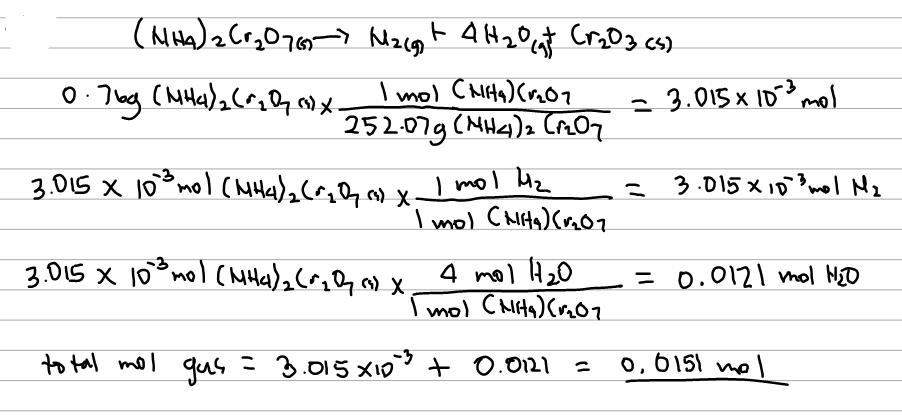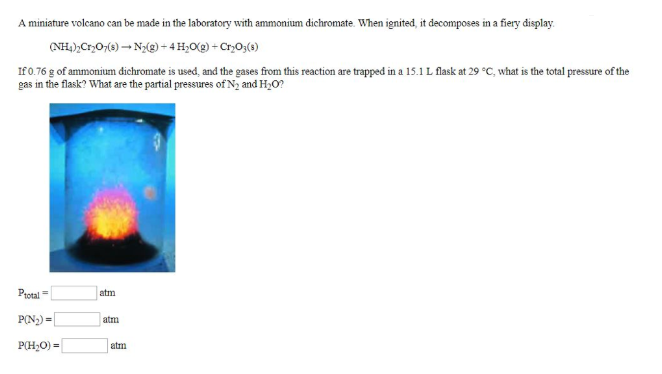# Problem: A miniature volcano can be made in the laboratory with ammonium dichromate. When ignited, it decomposes in a fiery display. (NH4)2Cr2O7 (s) → N2 (g) + 4H2O (g) + Cr2O3 (s) If 0.76 g of ammonium dichromate is used, and the gases from this reaction are trapped in a 15.1 L flask at 29°C, what is the total pressure of the gas in the flask? What are the partial pressures of N2 and H2O?

###### FREE Expert Solution99% (190 ratings)###### Problem Details

A miniature volcano can be made in the laboratory with ammonium dichromate. When ignited, it decomposes in a fiery display.

(NH4)2Cr2O7 (s) → N(g) + 4H2O (g) + Cr2O3 (s)

If 0.76 g of ammonium dichromate is used, and the gases from this reaction are trapped in a 15.1 L flask at 29°C, what is the total pressure of the gas in the flask? What are the partial pressures of N2 and H2O?